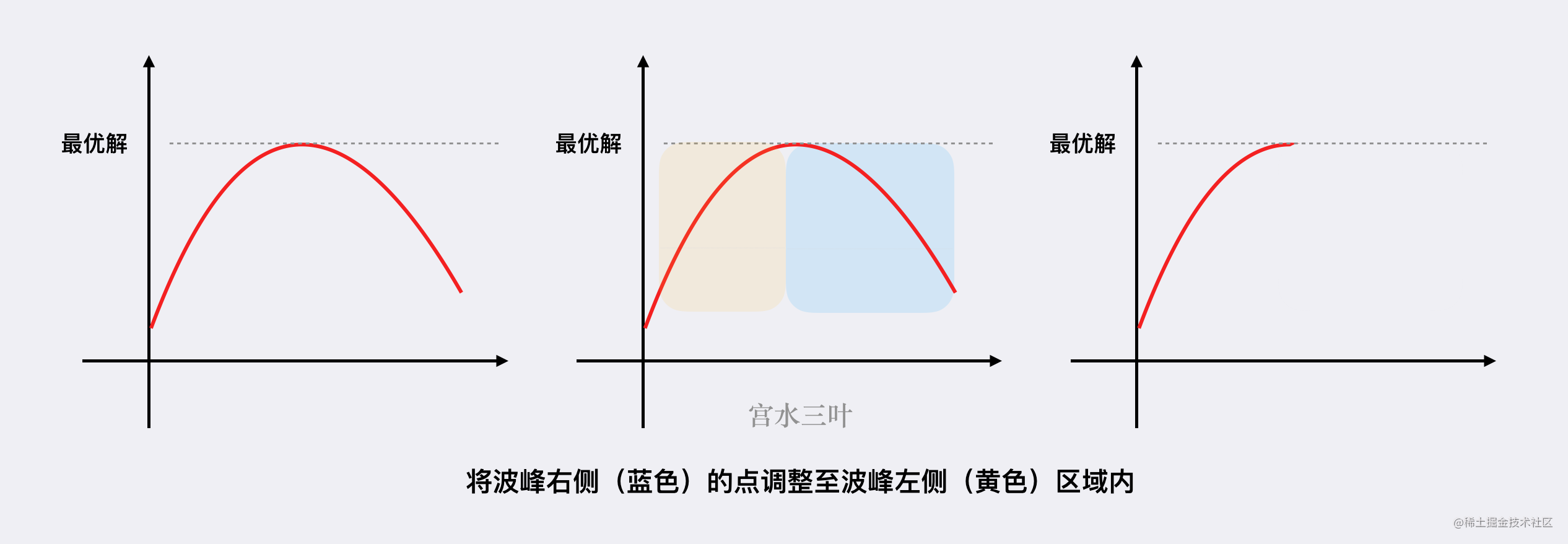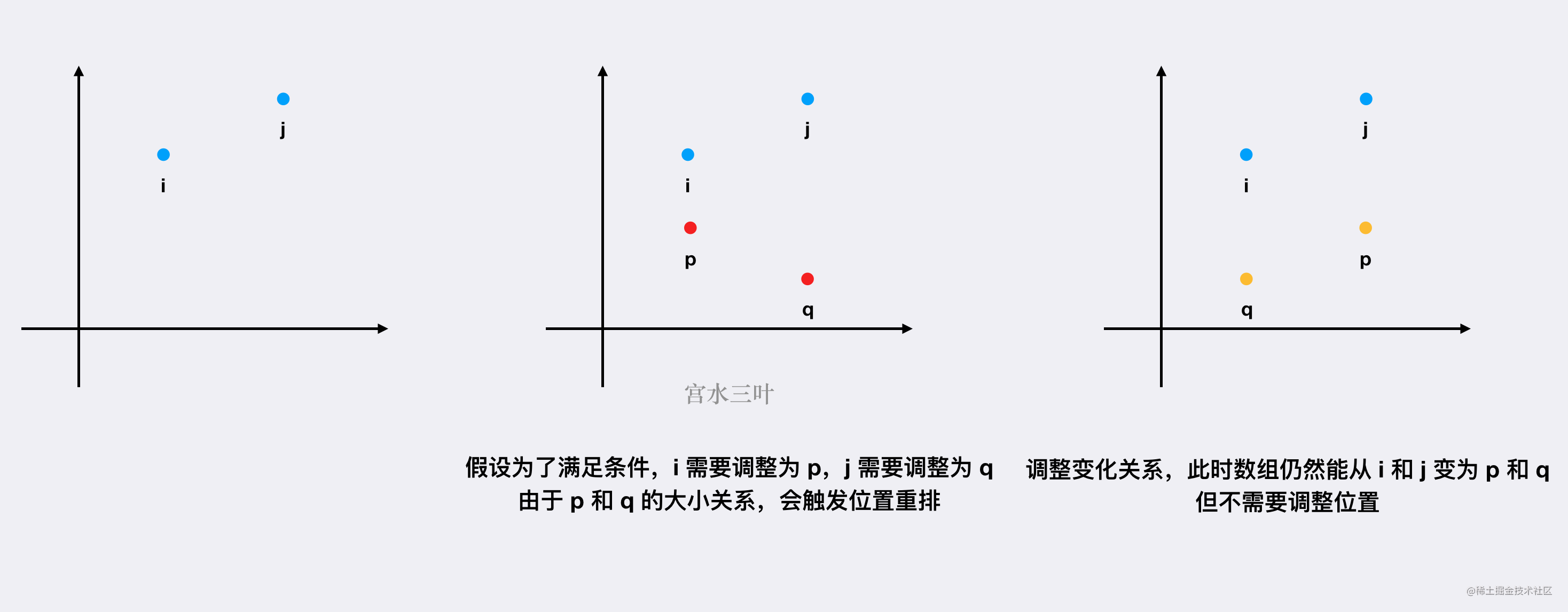# 【每日算法】贪心决策原排序数组的每一位，以及贪心解的正确性证明｜Python 主题月

## 题目描述

Tag : 「贪心」

• arr 中 第一个 元素必须为 1 。
• 任意相邻两个元素的差的绝对值 小于等于 1 ，也就是说，对于任意的 1 <= i < arr.length （数组下标从 0 开始），都满足 abs(arr[i] - arr[i - 1]) <= 1 。abs(x) 为 x 的绝对值。

• 减小 arr 中任意元素的值，使其变为一个 更小的正整数 。
• 重新排列 arr 中的元素，你可以以任意顺序重新排列。

输入：arr = [2,2,1,2,1]

arr 中最大元素为 2 。

输入：arr = [100,1,1000]

1. 重新排列 arr 得到 [1,100,1000] 。
2. 将第二个元素减小为 2 。
3. 将第三个元素减小为 3 。

arr 中最大元素为 3 。

输入：arr = [1,2,3,4,5]

• 1 <= arr.length <= $10^5$

1 <= arr[i] <= $10^9$

## 基本分析 & 证明

• （非严格）单调递减
• 存在波段
• （非严格）单调递增

• （非严格）单调递减：题目限定了数的范围为正整数，且第一位为 $1$，这种情况不用讨论了，跳过；
• 存在波段：我们始终可以将波峰的右侧出现的值，纳入到波峰的左侧，从而消掉这个波峰，最终将整个分布调整为「（非严格）单调递增」的形式，结果不会变差：b 不会比 a 更优。## 贪心

Java 代码：

class Solution {
public int maximumElementAfterDecrementingAndRearranging(int[] arr) {
int n = arr.length;
Arrays.sort(arr);
arr = 1;
for (int i = 1; i < n; i++) {
if (arr[i] - arr[i - 1] > 1) {
arr[i] = arr[i - 1] + 1;
}
}
return arr[n - 1];
}
}

Python 3 代码：

class Solution:
def maximumElementAfterDecrementingAndRearranging(self, arr: List[int]) -> int:
n = len(arr)
arr.sort()
arr = 1
for i in range(1, n):
if arr[i] - arr[i - 1] > 1:
arr[i] = arr[i - 1] + 1
return arr[n - 1]

• 时间复杂度：假定 Arrays.sort 使用的是双轴快排实现。复杂度为 $O(n\log{n})$
• 空间复杂度：假定 Arrays.sort 使用的是双轴快排实现。复杂度为 $O(\log{n})$